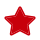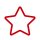Updated on 2023-03-14 GMT+08:00

# Binary Subtraction Operation

#### Function Name

\$subtract(intA, intB)

#### Function Description

Implements the subtraction operation between parameter A and parameter B, which support the following types:

• Numbers
• Environment parameters
• Local parameters
• Binary operations

#### Application Scenarios

The binary subtraction function can be used in the following scenarios for API automation:

• Request URL
• Request body
• Checkpoint property
• if condition
• for loop interrupt condition

#### Example

• Request URL

As shown in the following figure, the value of the test parameter in the request URL is the binary subtraction function. Parameters A and B in the function are 1001 and 1000 respectively.As shown in the following figure, the value of parameter subtract in the request header is the binary subtraction function. Parameter A in the function is the binary multiplication function \$multiply(100,100), and parameter B is -1.• Request body

As shown in the following figure, the binary subtraction function is used in the request body. Parameter A in the function is the binary division function \$divide (1000,100), and parameter B is the binary addition function \$add (1000,1000).• Checkpoint property

As shown in the following figure, the target value of the checkpoint property result is the binary subtraction function. Parameter A in the function is the local parameter test, and parameter B is 1. For details about how to set local parameters, see Local Parameters.• if condition

As shown in the following figure, the target value of the if condition is the binary subtraction function. Parameter A in the function is 1001, and parameter B is the environment variable status. For details about how to set environment parameters, see Setting Environment Parameters.• for loop interrupt condition

As shown in the following figure, the target value of the for loop interrupt condition is the binary subtraction function. Parameters A and B in the function are 1001 and 1000 respectively.